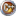# Case study: Goal Seeking

Introduction: Using MtxVec optimization routines you can with just few lines of code do unconstrained minimization, bounded minimization on scalar or vector function, all kinds of non-linear regressions, zero finding and even goal seeking (what-if) scenarios. This example uses financial functions to calculate monthly payments if we know the desired investment value we want to have at the end of payment. The notation used is payments from us are negative, payments to us are positive.

Case: The example follows the Excel goal-seeking examples, available in help files. Hypothetical what-if scenario is as follows: Let us say we want to save some money on monthly basis for four years and have 30.000 EIR by the end of the saving time (4 years, at 6% APR). What we want to know is how big should be monthly payment to reach our goal of 20.000 EUR. What we need is a function describing all this. Following a simple logic and geometic seriers sum value formula, we arrive at:where fv is future value (the sum we want in the future), rate is period rate, ppm monthly payment, cv present i.e current value of the investment and numper number of periods we want to invest money. In formula the assumption made is payments are always made at the end of the period. If not, we must multiply ppm term with additional (1+rate) term.

In our case the problem translates to:

1. Express fv as function of rate , numnper, ppm and cv.
2. Assume rate, numper and cv are fixed. Set values to: rate=6%/12, numper=48, cv=0 (no additional deposit at start), ppm=-200 (conservative initial estimate for monthly payment).
3. Set desired future value to 20.000. When doing minimization, the goal will be to minimize square of difference between fv and desired future value.
4. Set parameters lower and upper bounds. The only variing parameter is ppm with (-INF, -100). All other parameters lower and upper bounds are set to the initial values: rate [0.005,0.005], numper[48,48], cv[0,0].
5. Use Simplex method to get optimal value for ppm meaning if you insert this value into fv formula, you will end up with fv value 20.000.``````uses MtxExpr, Optimization, MtxVec;

function FVObjFun(Parameters: TVec; const Constants: array of TSample;
OC: array of TObject): TSample;
var rate, pmt, pv, fv, tv, fac: TSample;
begin
// All payments are made at the end of the period!
rate := Parameters; // rate
numper := Round(Parameters); // number of periods
pmt := Parameters; // payment per period
pv := Parameters; // lump present value, default 0.00
tv := Constants; // desired future value
fac := IntPower(1+rate,nuimper);
fv := -(pv*fac + pmt*(fac-1)/rate); // future value
// minimizing square of difference, not difference.
Result := Sqr(fv - tv);
end;

procedure DoCalculation;
var stopReason: TOptStopReason;
targetValue, rss: TSample;
numper: Integer;
pars; array[0..3] of TSample;
begin
pars := 0.06/12;
pars := 48;
pars := -200;
pars := 0.0;

Simplex(FVObjFun, pars, [targetValue], nil,
[0.005,48,-INF,0.0], [0.005,48,-50,0.0],
rss, stopReason, 500, 1e-4, nil);
end;````````````using System;
using System.Collections.Generic;
using System.Text;
using Dew.Math;
using Dew.Math.Units;

namespace Dew.Examples
{
class Program
{

static double FVObjFun(TVec Parameters, double[] Constants,
params object[] ObjConst)
{
// All payments are made at the end of the period!
double rate = Parameters; // rate
int periods = Convert.ToInt16(Parameters); // number of periods
double pmt = Parameters; // payment per period
double pv = Parameters; // lump present value, default 0.00
double tv = Constants;
double fac = Math387.IntPower(1+rate,periods);
double fv = -(pv*fac + pmt*(fac-1)/rate); // future value

// minimizing square of difference, not difference.
return (fv - tv) * (fv - tv);
}

static void DoCalculation()
{
TOptStopReason stopReason;
double rss;
double targetValue = 20000; // desired fv

double[] pars = new double;
pars = 0.06/12; // APR 6%, monthly rate 0.5% - fixed
pars = 48; // months i.e number of periods - fixed
pars = -200; // period payment- VARIING parameter
pars = 0.0; // lump payment i.e. present value = 0 - fixed
// Output to console
Console.WriteLine("");
Console.WriteLine("Goal seeking - Case study 005 - C#\n");
Console.WriteLine("Monthly period rate:\t"
+ pars.ToString("0.00%"));
Console.WriteLine("Periods(months):\t" +
Convert.ToInt16(pars).ToString());
Console.WriteLine("payment per period:\t"
+ pars.ToString("0.00"));
Console.WriteLine("Desired future value:\t"
+ targetValue.ToString("0.00"));
// #1 vary only monthly payment, acceptable values are between -inf and -50.
// Other parameters are fixed.
Optimization.Simplex(FVObjFun, ref pars, new double { targetValue },
null,
new double {0.08/12,48,-Math387.INF,0},
new double {0.08/12,48,-100,0.0},
out rss, out stopReason, 500, 1e-4, null);
Console.WriteLine("----------------------------------------------");
Console.WriteLine("If we are to have " + targetValue.ToString()
+ " by the end of the investment,");
Console.WriteLine("then the payment per period should be "
+ pars.ToString("0.00") + "\n");
Console.Read();
}

static void Main(string[] args)
{
DoCalculation();
}
}
}``````

The result is -354.93 EUR: if you make a monthly deposit 354.93 EUR at 6% APR for 4 years, you'll have 20,000 EUR by the end of the saving period. Result from Excel is the same.

Additional things to try:

• Try variing Rate parameter while keeping monthly payment fixed.
• Run goal seeking in Excel and compare the results with MtxVec.
• Express ppm or cv from the above formula and calculate optimal future value or monthly payment.

### Information

© DewResearch 1997 - 2020 All Rights Reserved.

E-mail This email address is being protected from spambots. You need JavaScript enabled to view it..
Delphi & C++ Builder are registered trademarks of Embarcadero Corporation. All other brands and product names are trademarks or registered trademarks of their respective owners.